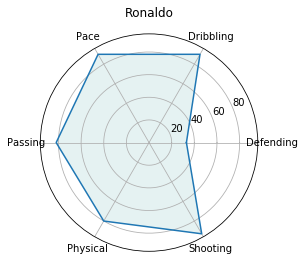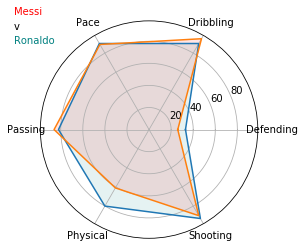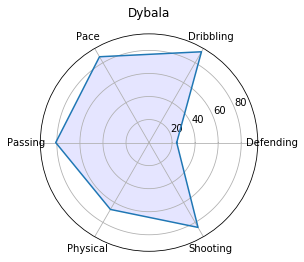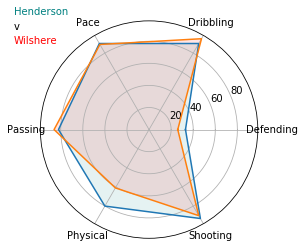In football analysis and video games, radar charts have been popularised in a number of places, from the FIFA series, to Ted Knutson’s innovative ways of displaying player data.

Radar charts are an engaging way to show data that typically piques more attention than a bar chart although you can often use both of these to show the same data.

This article runs through the creation of basic radar charts in Matplotlib, plotting the FIFA Ultimate Team data of a couple of players, before creating a function to streamline the process. To start, let’s get our libraries and data pulled together.

In :
```import pandas as pd
from math import pi
import matplotlib.pyplot as plt
%matplotlib inline

#Create a data frame from Messi and Ronaldo's 6 Ultimate Team data points from FIFA 18
Messi = {'Pace':89,'Shooting':90,'Passing':86,'Dribbling':95,'Defending':26,'Physical':61}
Ronaldo = {'Pace':90,'Shooting':93,'Passing':82,'Dribbling':90,'Defending':33,'Physical':80}

data = pd.DataFrame([Messi,Ronaldo], index = ["Messi","Ronaldo"])
data
```
Out:
Defending Dribbling Pace Passing Physical Shooting
Messi 26 95 89 86 61 90
Ronaldo 33 90 90 82 80 93

Plotting data in a radar has lots of similarities to plotting along a straight line (like a bar chart). We still need to provide data on where our line goes, we need to label our axes and so on. However, as it is a circle, we will also need to provide the angle at which the lines run. This is much easier than it sounds with Python.

Firstly, let’s do the easy bits and take a list of Attributes for our labels, along with a basic count of how many there are.

In :
```Attributes =list(data)
AttNo = len(Attributes)
```

We then take a list of the values that we want to plot, then copy the first value to the end. When we plot the data, this will be the line that the radat follows – take a look below:

In :
```values = data.iloc.tolist()
values += values [:1]
values
```
Out:
`[33, 90, 90, 82, 80, 93, 33]`

So these are the point that we will draw on our radar, but we will need to find the angles between each point for our line to follow. The formula below finds these angles and assigns them to ‘angles’. Then, just as above, we copy the first value to the end of our array to complete the line.

In :
```angles = [n / float(AttNo) * 2 * pi for n in range(AttNo)]
angles += angles [:1]
```

Now that we have our values to plot, and the angles between them, drawing the radar is pretty simple.

Follow along with the comments below, but note the ‘polar=true’ in our subplot – this changes our chart from a more-traditional x and y axes chart, to a the circular radar chart that we are looking for.

In :
```ax = plt.subplot(111, polar=True)

#Add the attribute labels to our axes
plt.xticks(angles[:-1],Attributes)

#Plot the line around the outside of the filled area, using the angles and values calculated before
ax.plot(angles,values)

#Fill in the area plotted in the last line
ax.fill(angles, values, 'teal', alpha=0.1)

#Give the plot a title and show it
ax.set_title("Ronaldo")
plt.show()
```### Comparing two sets of data in a radar chart

One additional benefit of the radar chart is the ability to compare two observations (or players, in this case), quite easily.

The example below repeats the above process for finding angles for Messi’s data points, then plots them both together.

In :
```#Find the values and angles for Messi - from the table at the top of the page
values2 = data.iloc.tolist()
values2 += values2 [:1]

angles2 = [n / float(AttNo) * 2 * pi for n in range(AttNo)]
angles2 += angles2 [:1]

#Create the chart as before, but with both Ronaldo's and Messi's angles/values
ax = plt.subplot(111, polar=True)

plt.xticks(angles[:-1],Attributes)

ax.plot(angles,values)
ax.fill(angles, values, 'teal', alpha=0.1)

ax.plot(angles2,values2)
ax.fill(angles2, values2, 'red', alpha=0.1)

#Rather than use a title, individual text points are added
plt.figtext(0.2,0.9,"Messi",color="red")
plt.figtext(0.2,0.85,"v")
plt.figtext(0.2,0.8,"Ronaldo",color="teal")
plt.show()
```### Creating a function to plot individual players

This is a lot of code if we want to create multiple charts. We can easily turn these charts into a function, which will do all the heavy lifting for us – all we will have to do is provide it with a player name and data that we want to plot:

In :
```def createRadar(player, data):
Attributes = ["Defending","Dribbling","Pace","Passing","Physical","Shooting"]

data += data [:1]

angles = [n / 6 * 2 * pi for n in range(6)]
angles += angles [:1]

ax = plt.subplot(111, polar=True)

plt.xticks(angles[:-1],Attributes)
ax.plot(angles,data)
ax.fill(angles, data, 'blue', alpha=0.1)

ax.set_title(player)
plt.show()
```
In :
```createRadar("Dybala",[24,91,86,81,67,85])
```And how about we do the same thing to compare two players?

In :
```def createRadar2(player, data, player2, data2):
Attributes = ["Defending","Dribbling","Pace","Passing","Physical","Shooting"]

data += data [:1]
data2 += data2 [:1]

angles = [n / 6 * 2 * pi for n in range(6)]
angles += angles [:1]

angles2 = [n / 6 * 2 * pi for n in range(6)]
angles2 += angles2 [:1]

ax = plt.subplot(111, polar=True)

#Create the chart as before, but with both Ronaldo's and Messi's angles/values
ax = plt.subplot(111, polar=True)

plt.xticks(angles[:-1],Attributes)

ax.plot(angles,values)
ax.fill(angles, values, 'teal', alpha=0.1)

ax.plot(angles2,values2)
ax.fill(angles2, values2, 'red', alpha=0.1)

#Rather than use a title, individual text points are added
plt.figtext(0.2,0.9,player,color="teal")
plt.figtext(0.2,0.85,"v")
plt.figtext(0.2,0.8,player2,color="red")
plt.show()
```
In :
```createRadar2("Henderson", [76,76,62,82,81,70],"Wilshere", [62,82,71,80,72,69])
```### Summary

Radar charts are an interesting way to display data and allow us to compare two observations quite nicely. In this article, we have used them to compare fictional FIFA players, but analysts have used this format very innovatively to display actual performance data in an engaging format.